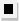Chapter 13.CR, Problem 57E### Algebra and Trigonometry (MindTap ...

4th Edition
James Stewart + 2 others
ISBN: 9781305071742

#### Solutions

Chapter
Section### Algebra and Trigonometry (MindTap ...

4th Edition
James Stewart + 2 others
ISBN: 9781305071742
Textbook Problem

# 5 5 - 6 0Infinite Geometric Series Determine whether the infinite geometric series is convergent or divergent. If it is convergent, find its sum. 5 − 5 ( 1.01 ) + 5 ( 1.01 ) 2 − 5 ( 1.01 ) 3 + ⋅ ⋅ ⋅

To determine

Whether the infinite geometric series 55(1.01)+5(1.01)25(1.01)3+ is convergent or divergent. Find the sum if convergent.

Explanation

Given:

The expression: 55(1.01)+5(1.01)25(1.01)3+

Approach:

Check if the absolute value of the common ratio is smaller than 1.

If convergent use the formula for sum of an infinite series.

Calculation:

The common ratio is,

### Still sussing out bartleby?

Check out a sample textbook solution.

See a sample solution

#### The Solution to Your Study Problems

Bartleby provides explanations to thousands of textbook problems written by our experts, many with advanced degrees!

Get Started